Home Practice
For learners and parents For teachers and schools
Textbooks
Full catalogue
Pricing SupportLog in

We think you are located in United States. Is this correct?

# 7.2 Right prisms and cylinders

## 7.2 Right prisms and cylinders (EMBHW)

A right prism is a geometric solid that has a polygon as its base and vertical sides perpendicular to the base. The base and top surface are the same shape and size. It is called a “right” prism because the angles between the base and sides are right angles.

A triangular prism has a triangle as its base, a rectangular prism has a rectangle as its base, and a cube is a rectangular prism with all its sides of equal length. A cylinder is another type of right prism which has a circle as its base. Examples of right prisms are given below: a rectangular prism, a cube, a triangular prism and a cylinder.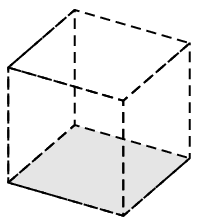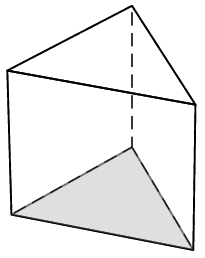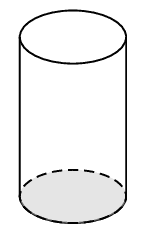### Surface area of prisms and cylinders (EMBHX)

Surface area is the total area of the exposed or outer surfaces of a prism. This is easier to understand if we imagine the prism to be a cardboard box that we can unfold. A solid that is unfolded like this is called a net. When a prism is unfolded into a net, we can clearly see each of its faces. In order to calculate the surface area of the prism, we can then simply calculate the area of each face, and add them all together.

For example, when a triangular prism is unfolded into a net, we can see that it has two faces that are triangles and three faces that are rectangles. To calculate the surface area of the prism, we find the area of each triangle and each rectangle, and add them together.

In the case of a cylinder the top and bottom faces are circles and the curved surface flattens into a rectangle with a length that is equal to the circumference of the circular base. To calculate the surface area we therefore find the area of the two circles and the rectangle and add them together.

Below are examples of right prisms that have been unfolded into nets. A rectangular prism unfolded into a net is made up of six rectangles.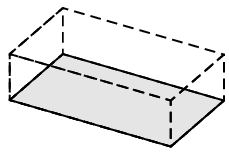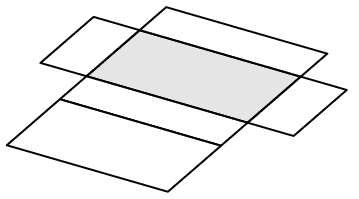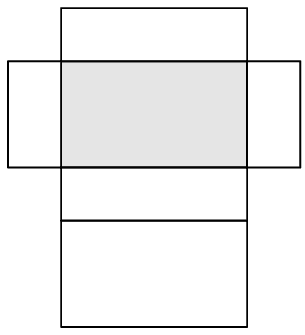A cube unfolded into a net is made up of six identical squares.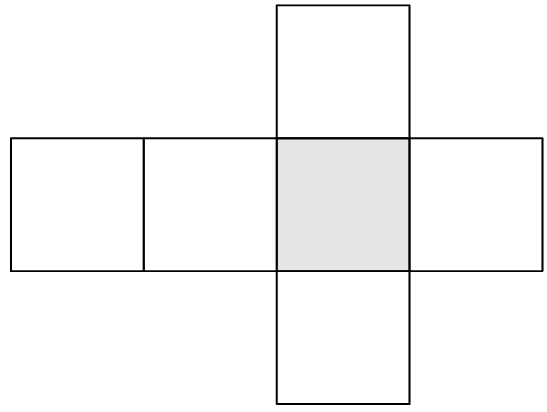A triangular prism unfolded into a net is made up of two triangles and three rectangles. The sum of the lengths of the rectangles is equal to the perimeter of the triangles.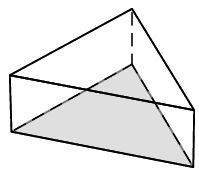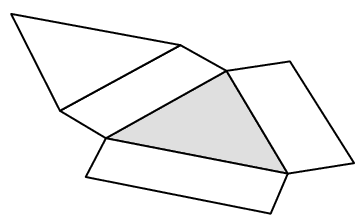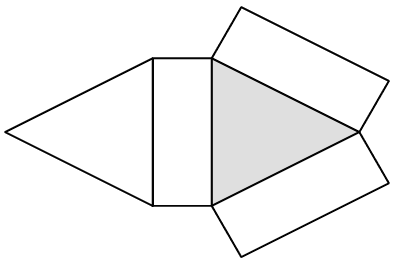A cylinder unfolded into a net is made up of two identical circles and a rectangle with length equal to the circumference of the circles.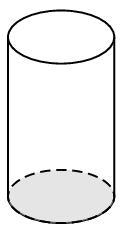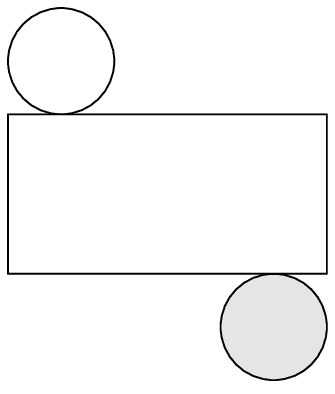temp text

## Worked example 2: Calculating surface area

A box of chocolates has the following dimensions:

\begin{align*} \text{length} &= \text{25}\text{ cm} \\ \text{width} &= \text{20}\text{ cm} \\ \text{height} &= \text{4}\text{ cm} \end{align*}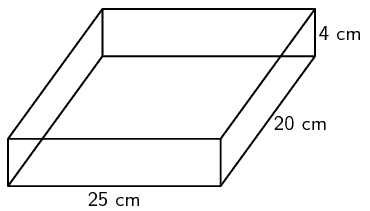And a cylindrical tin of biscuits has the following dimensions:

\begin{align*} \text{diameter} &= \text{20}\text{ cm} \\ \text{height} &= \text{20}\text{ cm} \end{align*}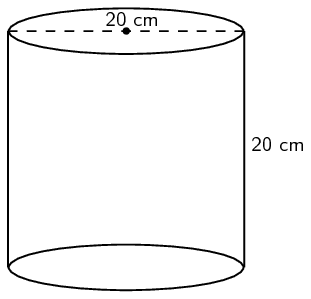1. Calculate the area of the wrapping paper needed to cover the entire box (assume no overlapping at the corners).
2. Determine if this same sheet of wrapping paper would be enough to cover the tin of biscuits.

### Determine the area of the rectangular box

\begin{align*} \text{Surface area} &= \text{2} \times (\text{25} \times \text{20}) + \text{2} \times (\text{20} \times \text{4}) + \text{2} \times (\text{25} \times \text{4}) \\ &= \text{1 360}\text{ cm$^{2}$} \end{align*}

### Determine the area of the cylindrical tin

The radius of the cylinder $$= \frac{\text{20}}{\text{2}} = \text{10}\text{ cm}$$.

\begin{align*} \text{Surface area} &= \text{2} \times \pi (\text{10})^2 + \text{2} \pi (\text{10})(\text{20}) \\ &= \text{1 885}\text{ cm$^{2}$} \end{align*}

No, the area of the sheet of wrapping paper used to cover the box is not big enough to cover the tin.

## Calculating surface area

Textbook Exercise 7.2

A popular chocolate container is an equilateral right triangular prism with sides of $$\text{34}$$ $$\text{mm}$$. The box is $$\text{170}$$ $$\text{mm}$$ long. Calculate the surface area of the box (to the nearest square centimetre).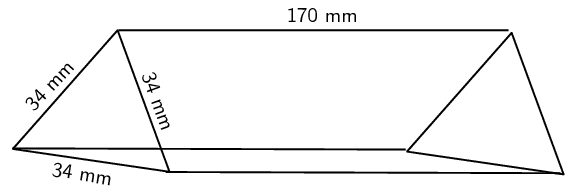\begin{align*} h&=\sin\text{60}\text{°} \\ \therefore h&=\text{29,4}\text{ mm} \\ \text{Area }\bigtriangleup &= 2\times\frac{1}{2}\times34\times\text{29,4} \\ &=\text{1 000}\text{ mm$^{2}$}\\ \text{Area rectangular sides} &=3\times170\times34 \\ &= \text{17 340}\text{ mm$^{2}$}\\ \text{Total surface area }&= \text{27 340}\text{ mm$^{2}$} \\ &= \text{273}\text{ cm$^{2}$} \end{align*}

Gordon buys a cylindrical water tank to catch rain water off his roof. He discovers a full $$\text{2}$$ $$\text{ℓ}$$ tin of green paint in his garage and decides to paint the tank (not the base). If he uses $$\text{250}$$ $$\text{ml}$$ to cover $$\text{1}$$ $$\text{m^{2}}$$, will he have enough green paint to cover the tank with one layer of paint?

Dimensions of the tank: \begin{align*} \text{diameter} &= \text{1,1}\text{ m} \\ \text{height} &= \text{1,4}\text{ m} \end{align*}\begin{align*} \text{Surface area }&=2\times\pi\times(\text{0,55})\times\text{1,4}+\pi(\text{0,55})^2 \\ &=\text{5,788}\text{ m$^{2}$} \\ \text{So, he needs } &\approx \text{6}\text{ m$^{2}$} \times \text{250}\text{ ml} \text{ paint} \\ &=\text{1 500}\text{ ml} \\ &= \text{1,5}\text{ ℓ} \end{align*}

Yes, he will have enough paint for $$\text{1}$$ layer.

### Volume of prisms and cylinders (EMBHY)

Volume, sometimes also called capacity, is the three dimensional space occupied by an object, or the contents of an object. It is measured in cubic units.

The volume of a right prism is simply calculated by multiplying the area of the base of a solid by the height of the solid.

 Rectangular prism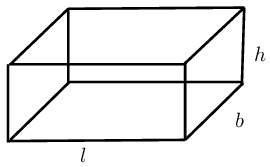\begin{aligned} \text{Volume} &= \text{area of base} \times \text{height} \\ &= \text{area of rectangle} \times \text{height} \\ &= l \times b \times h \end{aligned} Triangular prism\begin{aligned} \text{Volume} &= \text{area of base} \times \text{height} \\ &= \text{area of triangle} \times \text{height} \\ &= \left(\frac{1}{2}b\times h\right)\times H \end{aligned} Cylinder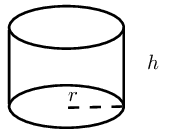\begin{aligned} \text{Volume} &= \text{area of base} \times \text{height} \\ &= \text{area of circle} \times \text{height} \\ & = \pi r^2 \times h \end{aligned}

## Worked example 3: Calculating volume

A rectangular glass vase with dimensions $$\text{28}\text{ cm} \times \text{18}\text{ cm} \times \text{8}\text{ cm}$$ is used for flower arrangements. A florist uses a platic cylindrical jug to pour water into the glass vase. The jug has a diameter of $$\text{142}$$ $$\text{mm}$$ and a height of $$\text{28}$$ $$\text{cm}$$.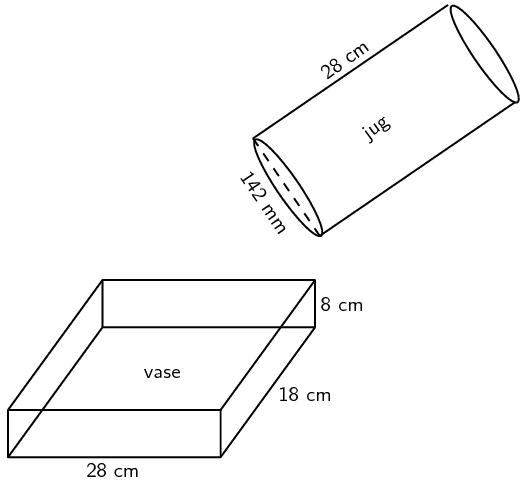1. Will the plastic jug hold $$\text{5}$$ $$\text{ℓ}$$ of water?

2. Will a full jug of water be enough to fill the glass vase?

### Determine the volume of the plastic jug

The diameter of the jug is $$\text{142}$$ $$\text{mm}$$, therefore the radius $$= \frac{\text{142}}{\text{2} \times \text{10}} = \text{7,1}\text{ cm}$$.

Volume of a cylinder = area of the base $$\times$$ height

\begin{align*} \text{Volume of the jug} &= \pi r^2 \times h \\ &= \pi \times (\text{7,1})^2 \times \text{28} \\ &= \text{4 434}\text{ cm$^{3}$} \\ \text{And }& \text{1 000}\text{ cm$^{3}$}= \text{1}\text{ ℓ} \\ \therefore \text{Volume of the jug} &= \frac{\text{4 434}}{\text{1 000}} \\ &= \text{4,434}\text{ ℓ} \end{align*}

No, the capacity of the jug is not enough to hold $$\text{5}$$ $$\text{ℓ}$$ of water.

### Determine the volume of the glass vase

Volume of a rectangular prism = area of the base $$\times$$ height

\begin{align*} \text{Volume of the vase} &= l \times b \times h \\ &= \text{28} \times \text{18} \times \text{8} \\ &= \text{4 032}\text{ cm$^{3}$} \\ \therefore \text{Volume of the vase} &= \frac{\text{4 032}}{\text{1 000}} \\ &= \text{4,032}\text{ ℓ} \end{align*}

Yes, the volume of the jug is greater than the volume of the vase.

temp text

## Calculating volume

Textbook Exercise 7.3

The roof of Phumza's house is the shape of a right-angled trapezium. A cylindrical water tank is positioned next to the house so that the rain on the roof runs into the tank. The diameter of the tank is $$\text{140}$$ $$\text{cm}$$ and the height is $$\text{2,2}$$ $$\text{m}$$.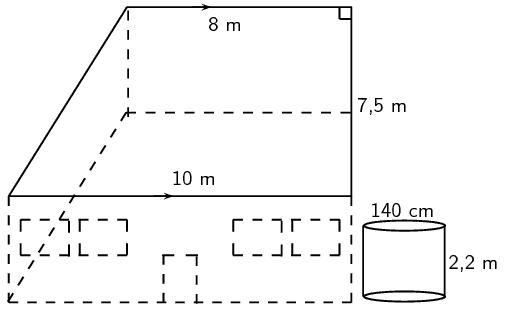Determine the area of the roof.

\begin{align*} \text{Area of roof } &=\frac{1}{2}(8+10) \times \text{7,5} \\ &=\text{67,5}\text{ m$^{2}$} \end{align*}
Determine how many litres of water the tank can hold.
\begin{align*} \text{Volume of tank}&= \pi(\text{0,7})^2\times \text{2,2}\\ &=\text{3,39}\text{ m$^{3}$} \\ &=\text{3,39}\text{ ℓ} \end{align*}

The length of a side of a hexagonal sweet tin is $$\text{8}$$ $$\text{cm}$$ and its height is equal to half of the side length.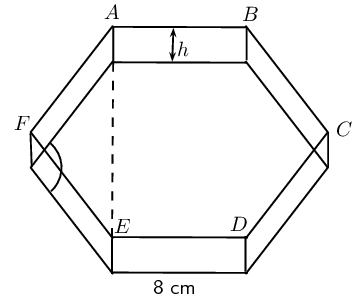Show that the interior angles are equal to $$\text{120}$$$$\text{°}$$.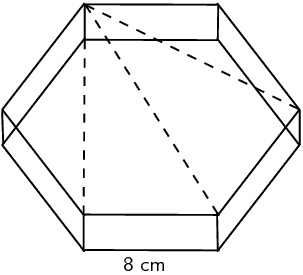Determine the length of the line $$AE$$.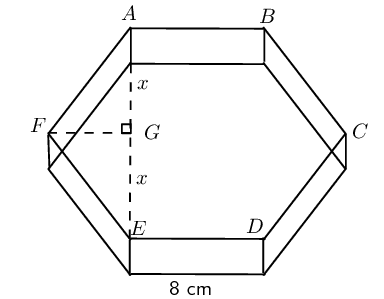Construct line $$FG$$ perpendicular to $$AE$$ such that $$G$$ lies on $$AE$$. Let $$AG = x$$ .

\begin{align*} AG = GE &= x \\ \text{In } \triangle AGF: \quad \frac{x}{8}&=\sin\text{60}\text{°} \\ \therefore x&= \text{6,93}\text{ cm} \\ \therefore AE &= 2 \times x \\ &= \text{13,86}\text{ cm} \end{align*}
Calculate the volume of the tin.
\begin{align*} \text{Volume } &= \text{area} \times h \\ &=(2\triangleleft 's+\square )\times 4\\ FG^2&=8^2-(8\sin\text{60}\text{°})^2 \\ \therefore FG &= \text{4}\text{ cm} \\ \text{OR} \\ \cos\text{60}\text{°}&= \frac{FG}{8} \\ \frac{1}{2} &= \frac{FG}{8} \\ \therefore FG &= \text{4}\text{ cm} \\ \text{Volume of box }&=\left [ 2\left ( \frac{1}{2} \times 8\sin\text{60}\text{°} \times 4 \right ) +(2(8\sin\text{60}\text{°}) \times 8)\right ]\times(4)\\ &= \text{138,56} \times 4 \\ &=\text{554,24}\text{ m$^{3}$} \end{align*}